Скачать презентацию Fixed capital Content: 1. Fixed Assets: general

fixed_capital12.ppt

• Размер: 697.5 Кб
• Количество слайдов: 71

## Описание презентации Fixed capital Content: 1. Fixed Assets: general по слайдам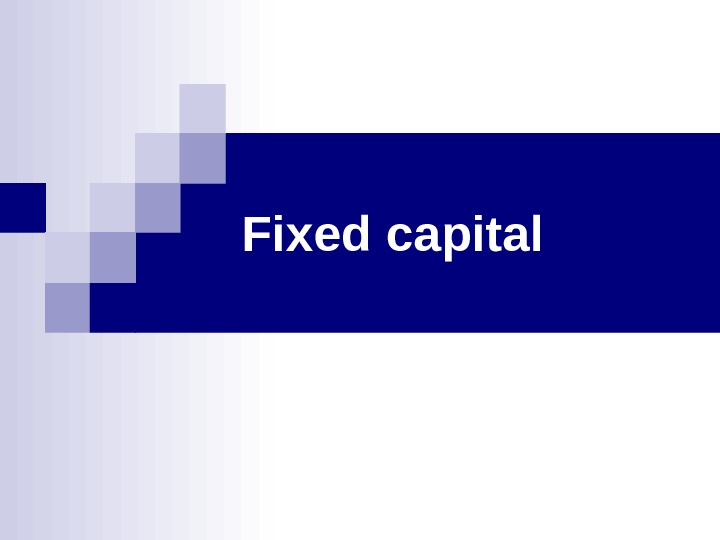Fixed capital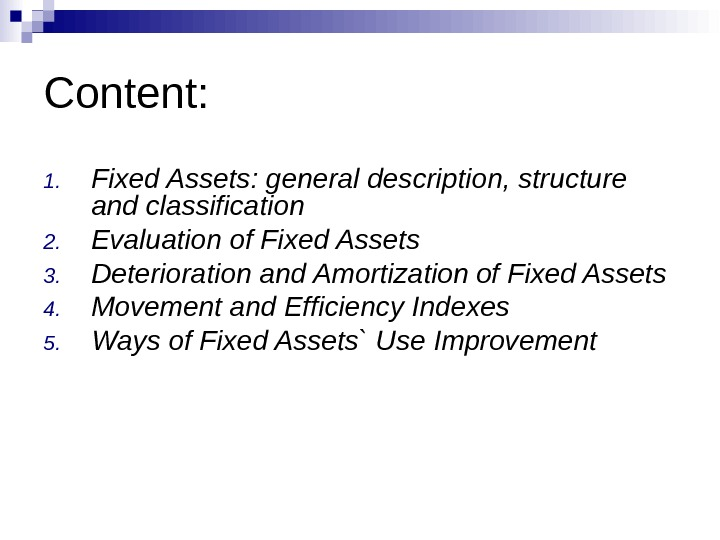Content: 1. Fixed Assets: general description, structure and classification 2. Evaluation of Fixed Assets 3. Deterioration and Amortization of Fixed Assets 4. Movement and Efficiency Indexes 5. Ways of Fixed Assets` Use Improvement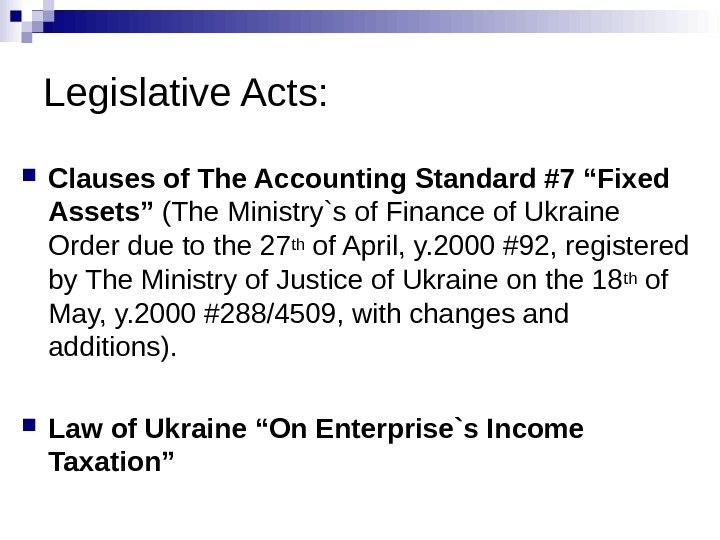Legislative Acts: Clauses of The Accounting Standard #7 “Fixed Assets” ( The Ministry`s of Finance of Ukraine Order due to the 27 th of April, y. 2000 #92, registered by The Ministry of Justice of Ukraine on the 18 th of May, y. 2000 # 288/4509, with changes and additions ). Law of Ukraine “On Enterprise`s Income Taxation”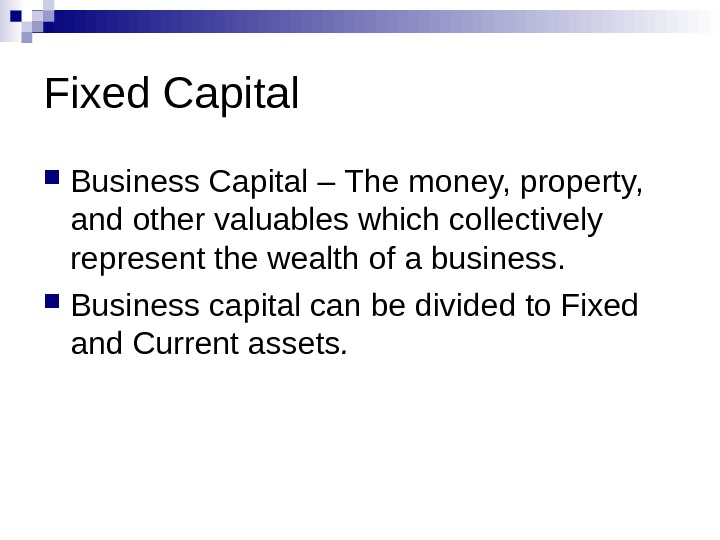Fixed Capital Business Capital – The money, property, and other valuables which collectively represent the wealth of a business. Business capital can be divided to Fixed and Current assets.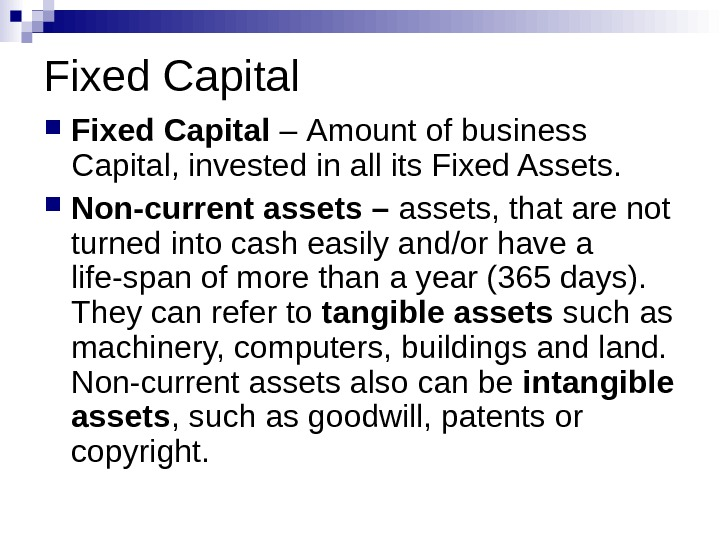Fixed Capital – Amount of business Capital, invested in all its Fixed Assets. Non-current assets – assets, that are not turned into cash easily and/or have a life-span of more than a year (365 days). They can refer to tangible assets such as machinery, computers, buildings and land. Non-current assets also can be intangible assets , such as goodwill, patents or copyright.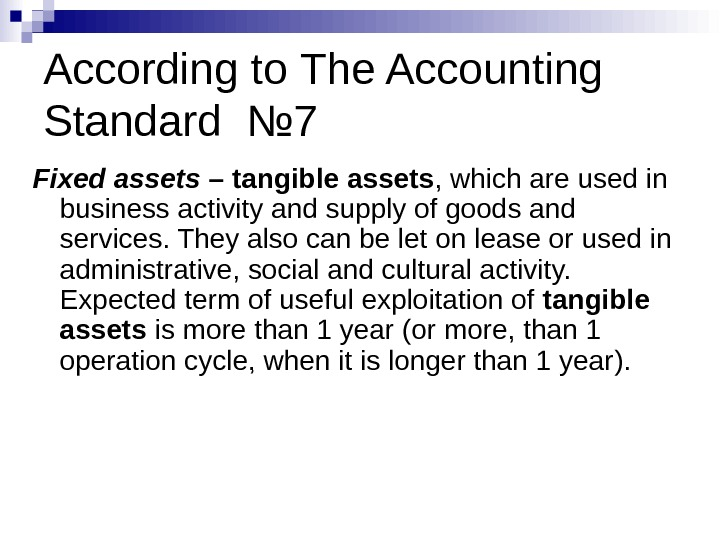According to The Accounting Standard № 7 Fixed assets – tangible assets , which are used in business activity and supply of goods and services. They also can be let on lease or used in administrative, social and cultural activity. Expected term of useful exploitation of tangible assets is more than 1 year (or more, than 1 operation cycle, when it is longer than 1 year ).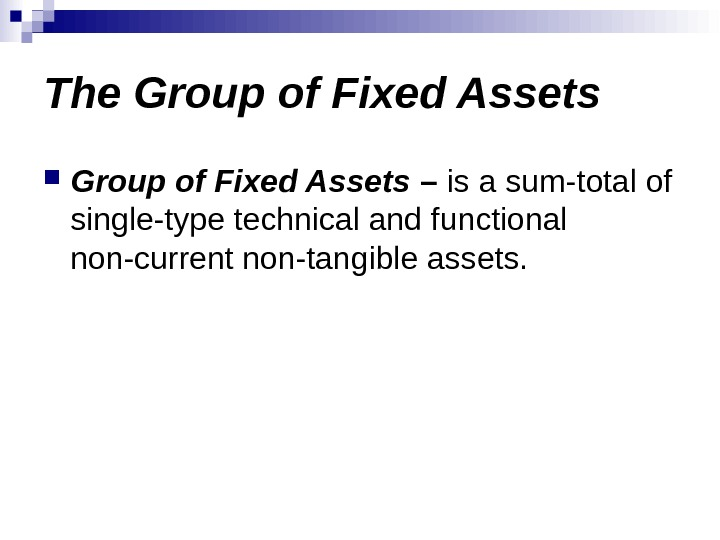The Group of Fixed Assets – is a sum-total of single-type technical and functional non-current non-tangible assets.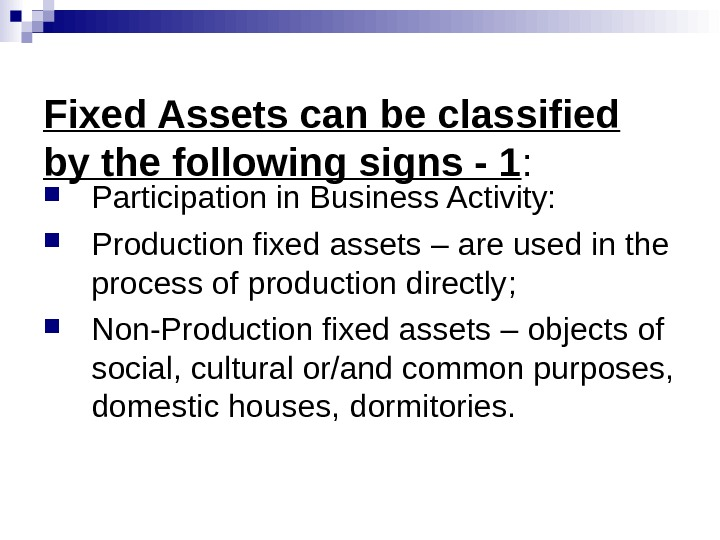Fixed Assets can be classified by the following signs — 1 : Participation in Business Activity: Production fixed assets – are used in the process of production directly ; Non-Production fixed assets – objects of social, cultural or/and common purposes, domestic houses, dormitories.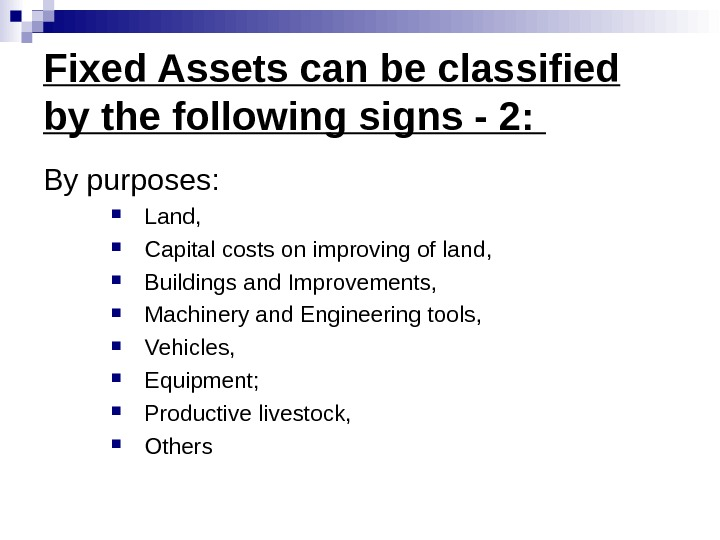Fixed Assets can be classified by the following signs — 2: By purposes : Land , Capital costs on improving of land , Buildings and Improvements, Machinery and Engineering tools, Vehicles, Equipment ; Productive livestock, Others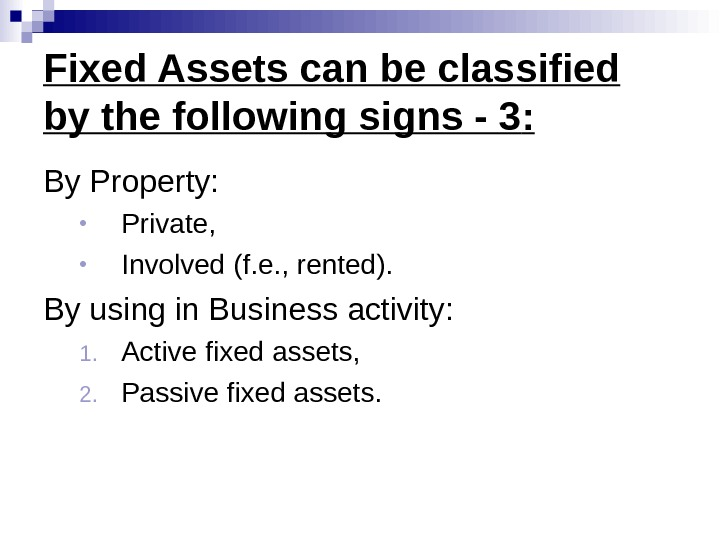Fixed Assets can be classified by the following signs — 3 : By Property : • Private, • Involved (f. e. , rented). By using in Business activity : 1. Active fixed assets, 2. Passive fixed assets.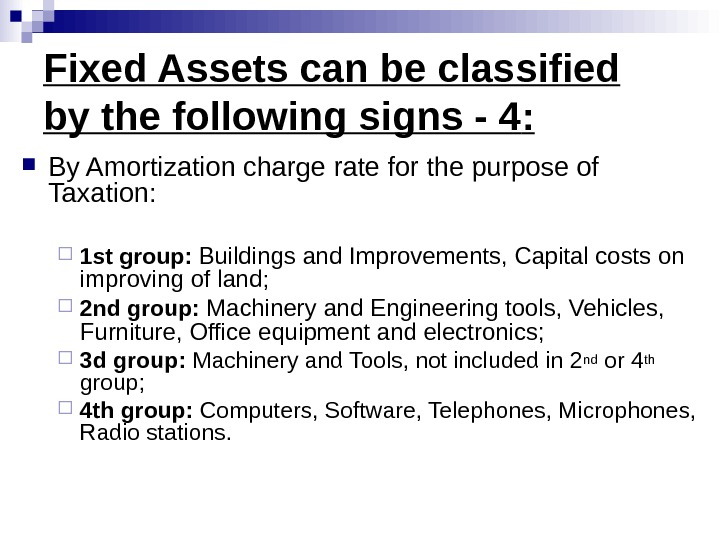Fixed Assets can be classified by the following signs — 4 : By Amortization charge rate for the purpose of Taxation : 1 st group : Buildings and Improvements, Capital costs on improving of land ; 2 nd group : Machinery and Engineering tools, Vehicles, Furniture, Office equipment and electronics; 3 d group : Machinery and Tools, not included in 2 nd or 4 th group ; 4 th group : Computers, Software, Telephones, Microphones, Radio stations.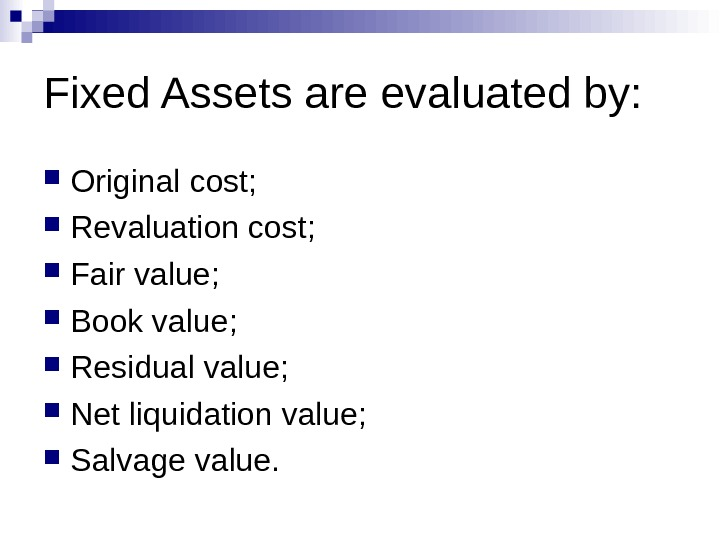Fixed Assets are evaluated by: Original cost ; Revaluation cost ; Fair value ; Book value ; Residual value; Net liquidation value; Salvage value.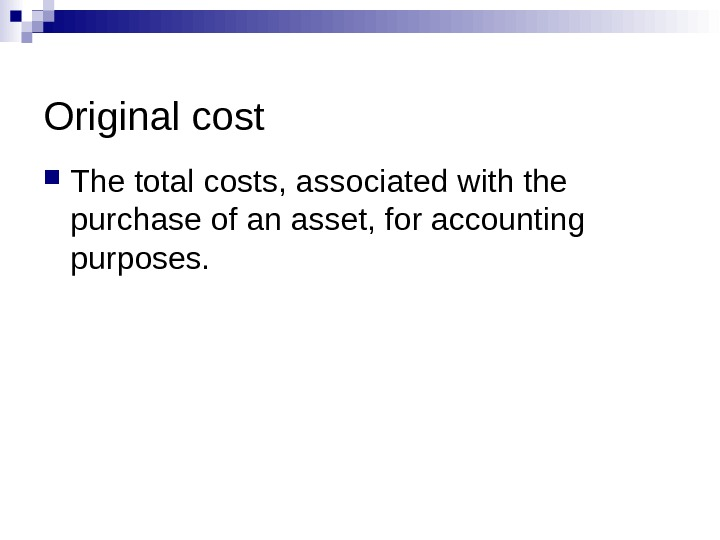Original cost The total costs, associated with the purchase of an asset, for accounting purposes.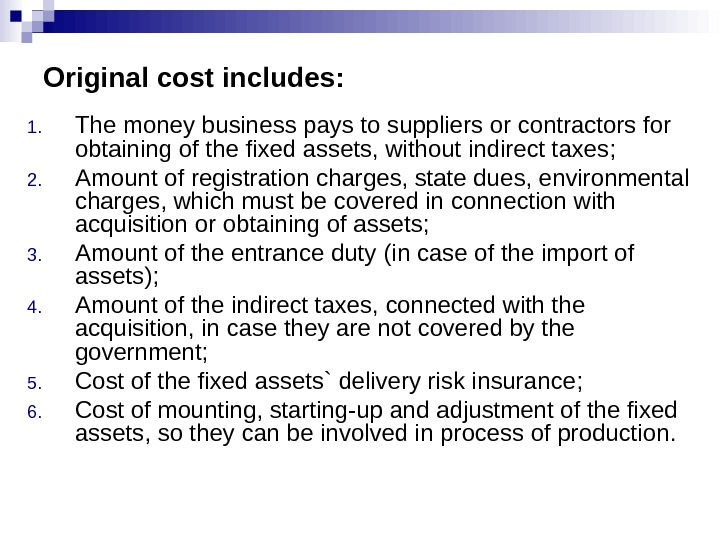Original cost includes: 1. The money business pays to suppliers or contractors for obtaining of the fixed assets, without indirect taxes ; 2. Amount of registration charges, state dues, environmental charges, which must be covered in connection with acquisition or obtaining of assets ; 3. Amount of the entrance duty ( in case of the import of assets ); 4. Amount of the indirect taxes, connected with the acquisition, in case they are not covered by the government; 5. Cost of the fixed assets` delivery risk insurance ; 6. Cost of mounting, starting-up and adjustment of the fixed assets, so they can be involved in process of production.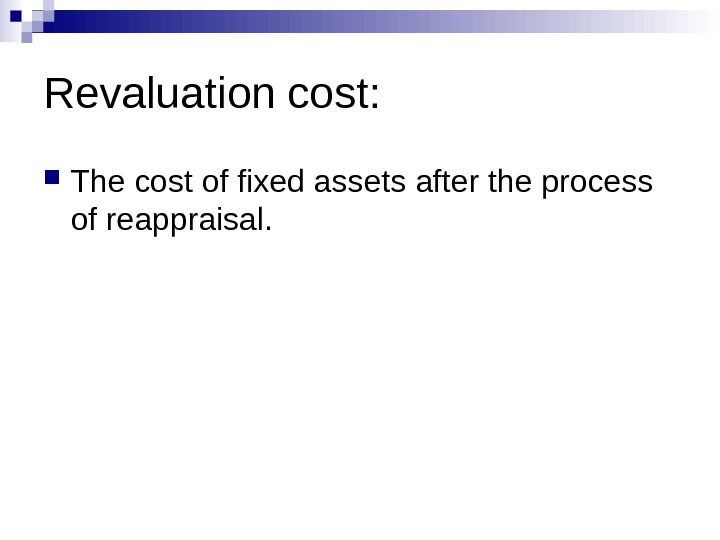Revaluation cost: The cost of fixed assets after the process of reappraisal.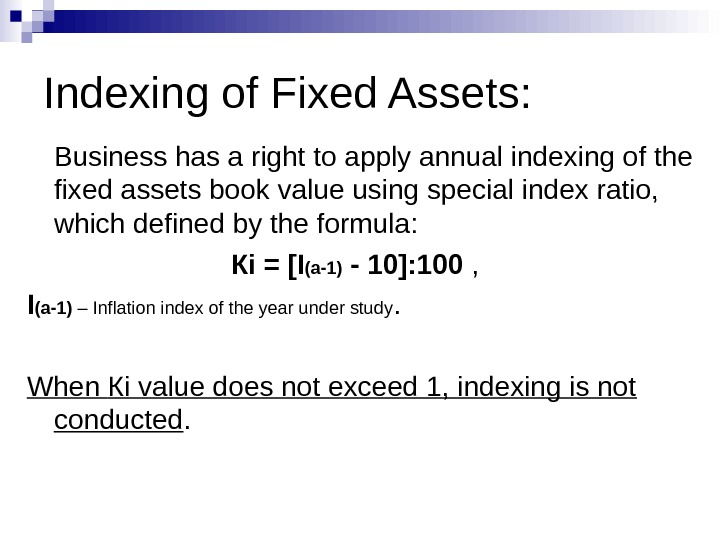Indexing of Fixed Assets: Business has a right to apply annual indexing of the fixed assets book value using special index ratio, which defined by the formula : Кі = [I (а-1) — 10]: 100 , I (а-1) – Inflation index of the year under study. When Кі value does not exceed 1, indexing is not conducted.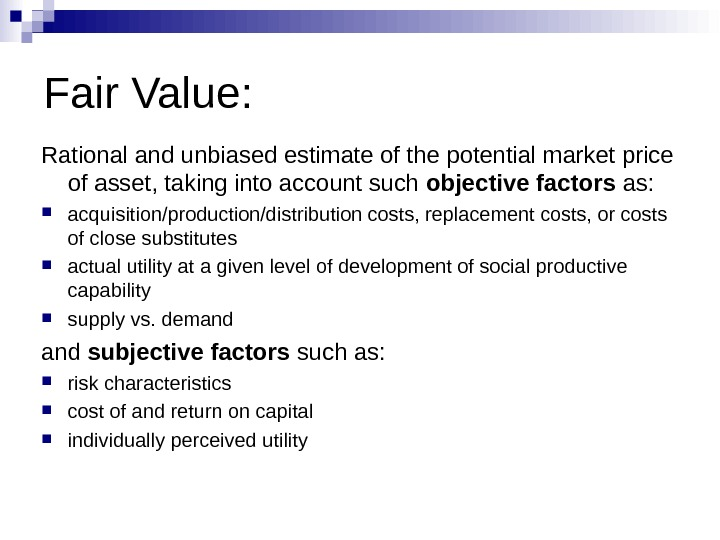Fair Value: Rational and unbiased estimate of the potential market price of asset, taking into account such objective factors as: acquisition/production/distribution costs, replacement costs, or costs of close substitutes actual utility at a given level of development of social productive capability supply vs. demand subjective factors such as: risk characteristics cost of and return on capital individually perceived utility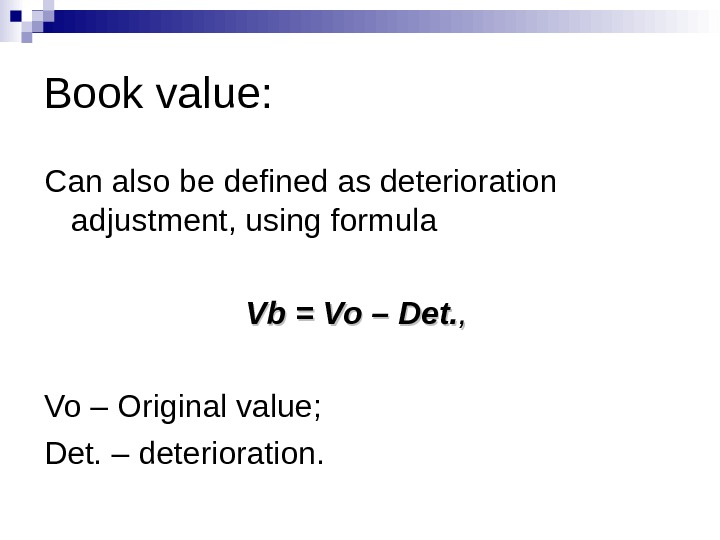Book value: Can also be defined as deterioration adjustment, using formula Vb. Vb = = Vo. Vo – – Det. , , Vo – Original value ; Det. – deterioration.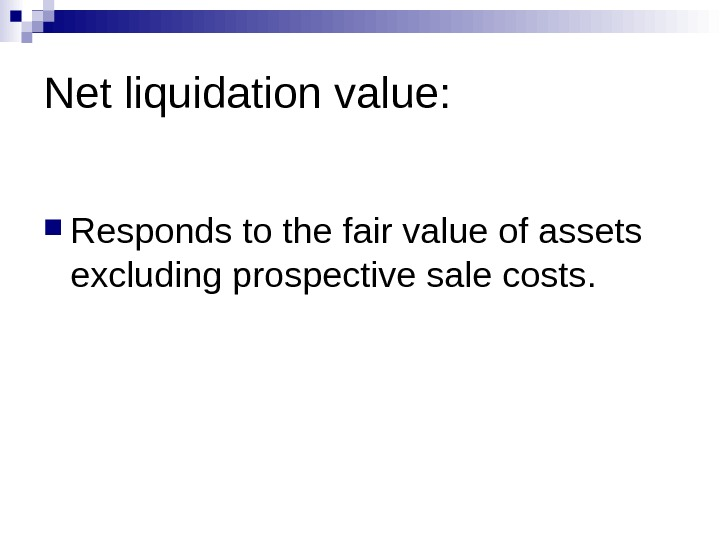Net liquidation value: Responds to the fair value of assets excluding prospective sale costs.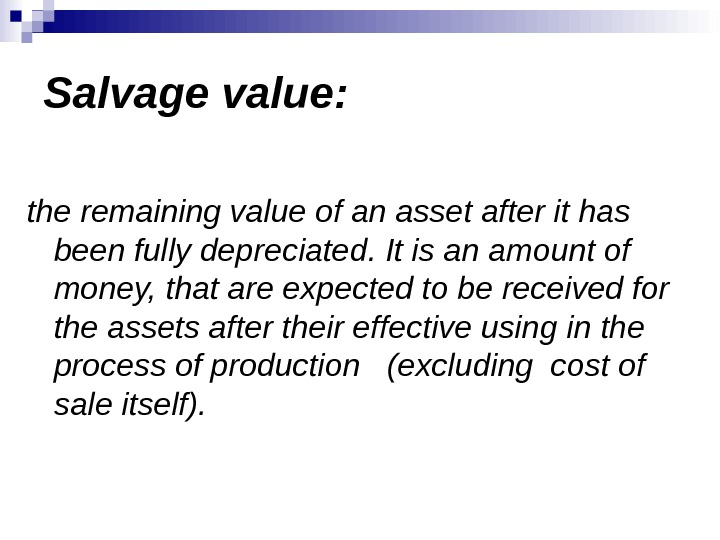Salvage value: the remaining value of an asset after it has been fully depreciated. It is an amount of money, that are expected to be received for the assets after their effective using in the process of production (excluding cost of sale itself).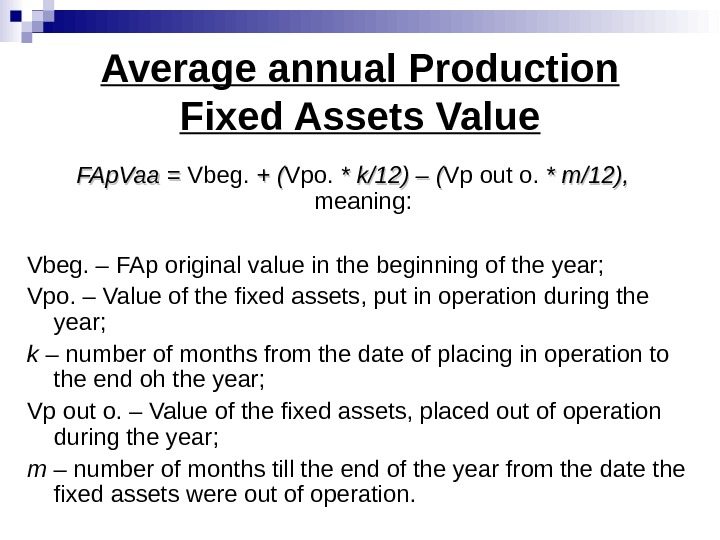Average annual Production Fixed Assets Value FAp. Vaa = = Vbeg. + ( Vpo. * * kk /12) – ( Vp out o. * * mm /12), meaning: Vbeg. – FAp original value in the beginning of the year; Vpo. – Value of the fixed assets, put in operation during the year; k – number of months from the date of placing in operation to the end oh the year; Vp out o. – Value of the fixed assets, placed out of operation during the year; m – number of months till the end of the year from the date the fixed assets were out of operation.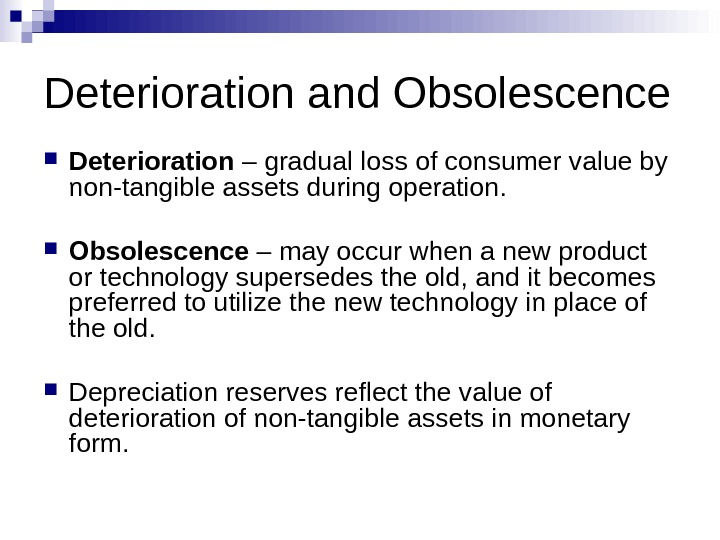Deterioration and Obsolescence Deterioration – gradual loss of consumer value by non-tangible assets during operation. Obsolescence – may occur when a new product or technology supersedes the old, and it becomes preferred to utilize the new technology in place of the old. Depreciation reserves reflect the value of deterioration of non-tangible assets in monetary form.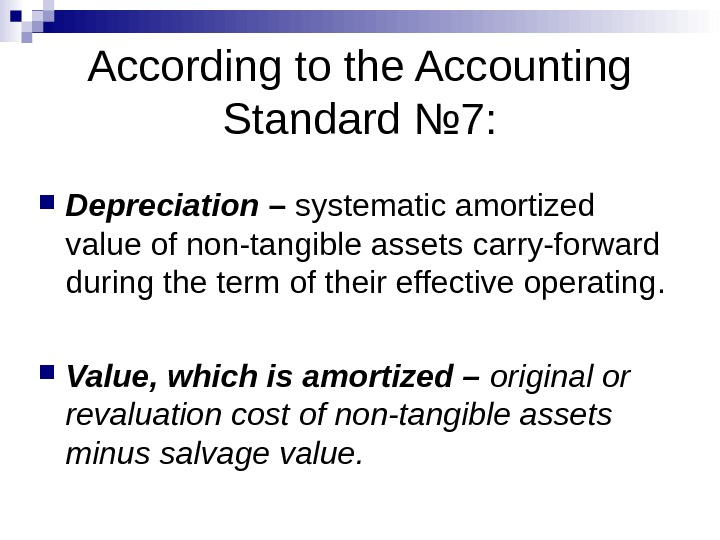According to the Accounting Standard № 7 : Depreciation – systematic amortized value of non-tangible assets carry-forward during the term of their effective operating. Value, which is amortized – original or revaluation cost of non-tangible assets minus salvage value.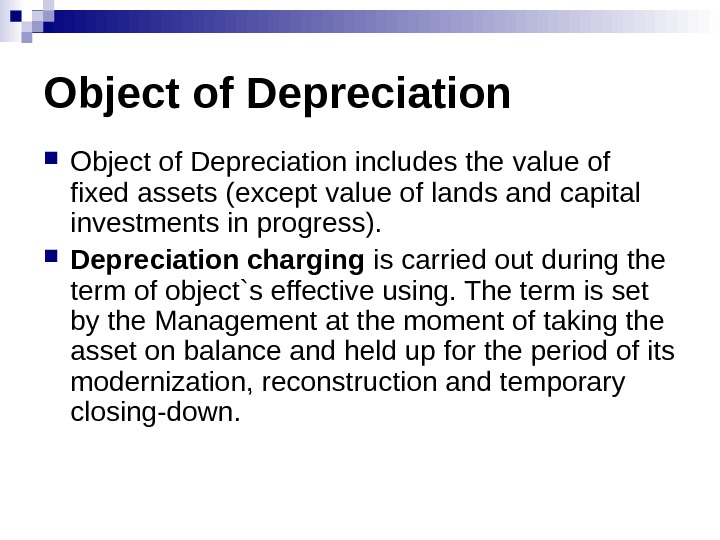Object of Depreciation includes the value of fixed assets (except value of lands and capital investments in progress). Depreciation charging is carried out during the term of object`s effective using. The term is set by the Management at the moment of taking the asset on balance and held up for the period of its modernization, reconstruction and temporary closing-down.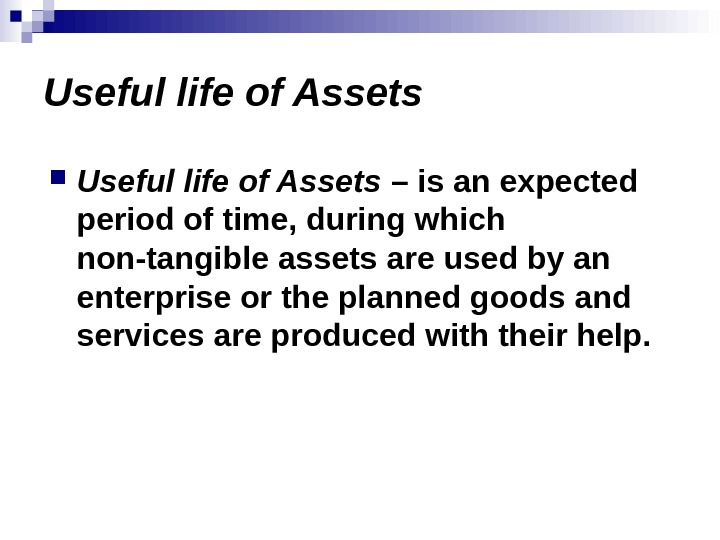Useful life of Assets – is an expected period of time, during which non-tangible assets are used by an enterprise or the planned goods and services are produced with their help.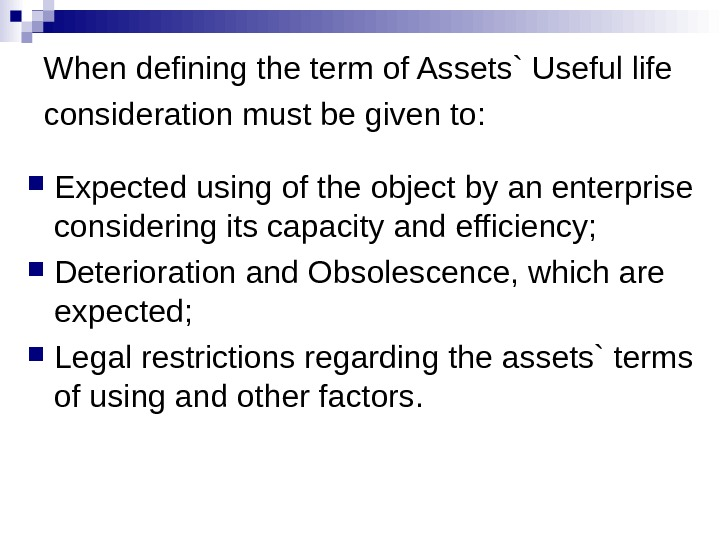When defining the term of Assets` Useful life consideration must be given to : Expected using of the object by an enterprise considering its capacity and efficiency; Deterioration and Obsolescence, which are expected ; Legal restrictions regarding the assets` terms of using and other factors.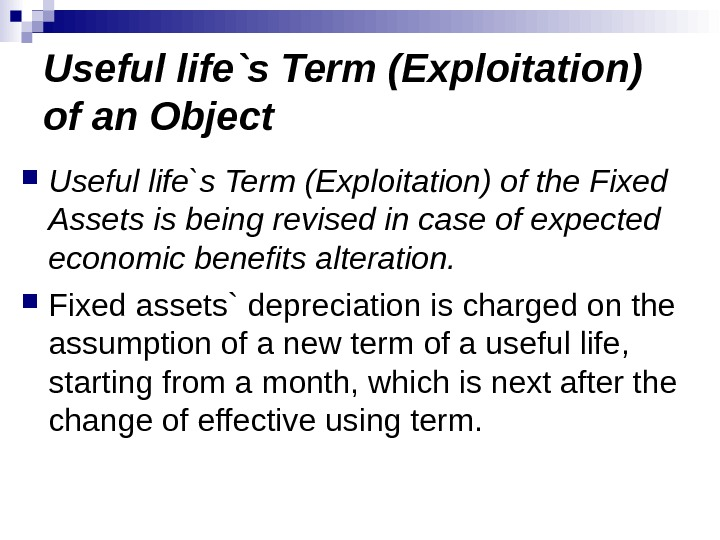Useful life`s Term (Exploitation) of an Object Useful life`s Term (Exploitation) of the Fixed Assets is being revised in case of expected economic benefits alteration. Fixed assets` depreciation is charged on the assumption of a new term of a useful life, starting from a month, which is next after the change of effective using term.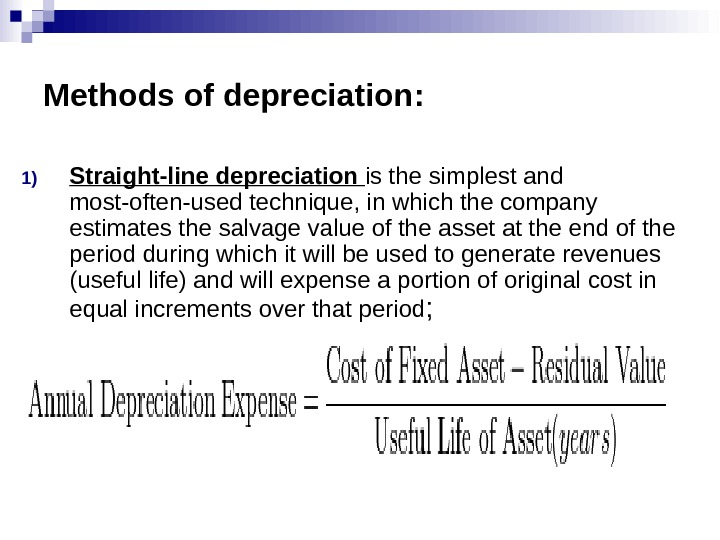Methods of depreciation : 1) Straight-line depreciation is the simplest and most-often-used technique, in which the company estimates the salvage value of the asset at the end of the period during which it will be used to generate revenues (useful life) and will expense a portion of original cost in equal increments over that period ;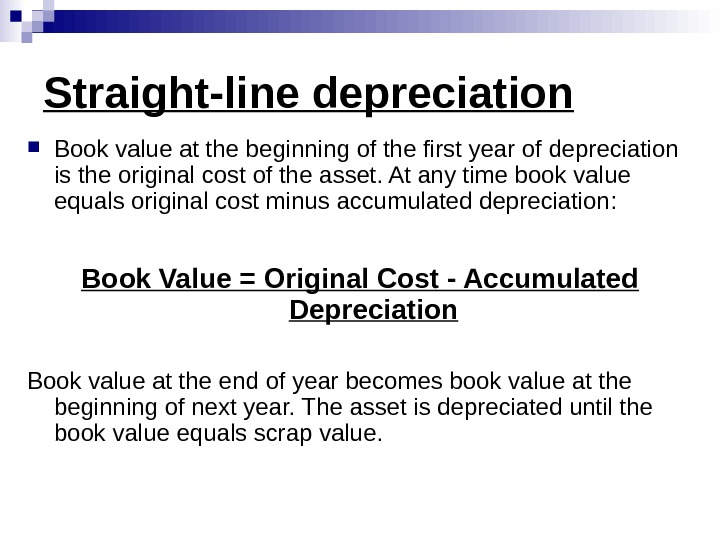Straight-line depreciation Book value at the beginning of the first year of depreciation is the original cost of the asset. At any time book value equals original cost minus accumulated depreciation : Book Value = Original Cost — Accumulated Depreciation Book value at the end of year becomes book value at the beginning of next year. The asset is depreciated until the book value equals scrap value.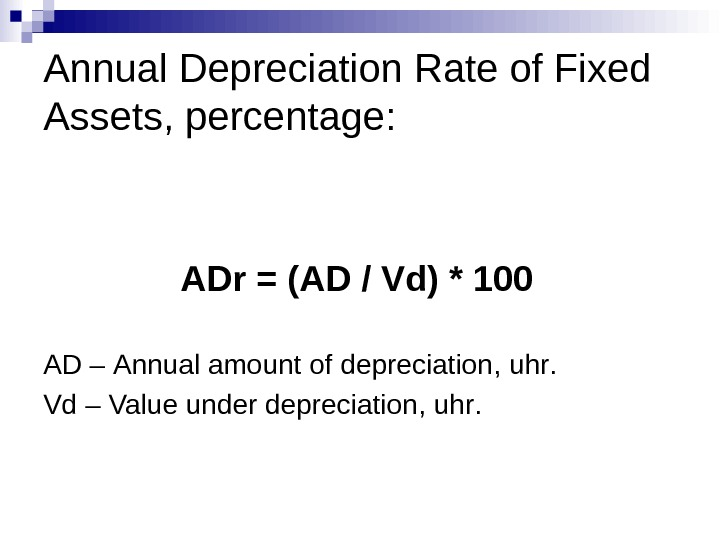Annual Depreciation Rate of Fixed Assets, percentage: ADr = ( AD / Vd ) * 100 AD – Annual amount of depreciation , uhr. Vd – Value under depreciation , uhr.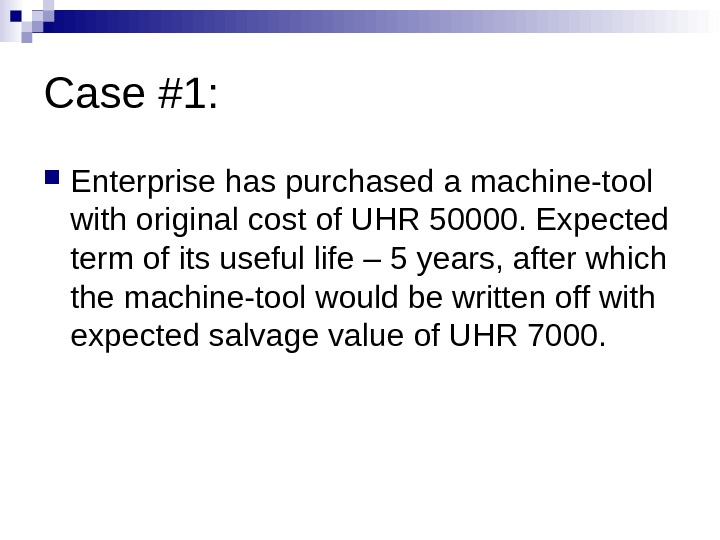Case #1: Enterprise has purchased a machine-tool with original cost of UHR 50000. Expected term of its useful life – 5 years, after which the machine-tool would be written off with expected salvage value of UHR 7000.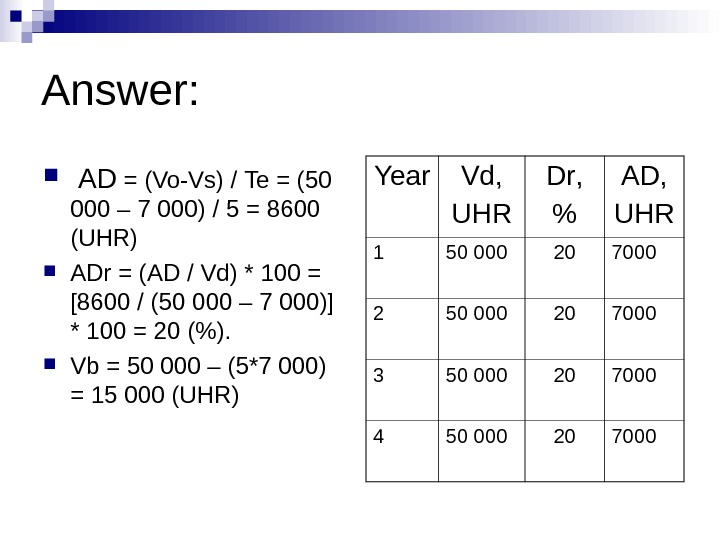Answer: AD = (Vo-Vs) / Te = ( 50 000 – 7 000) / 5 = 86 00 ( UHR ) ADr = ( AD / Vd ) * 100 = [8600 / ( 50 000 – 7 000) ] * 100 = 20 (%). Vb = 50 000 – ( 5 * 7 000) = 15 000 ( UHR ) Year Vd , UHR Dr , % AD , UHR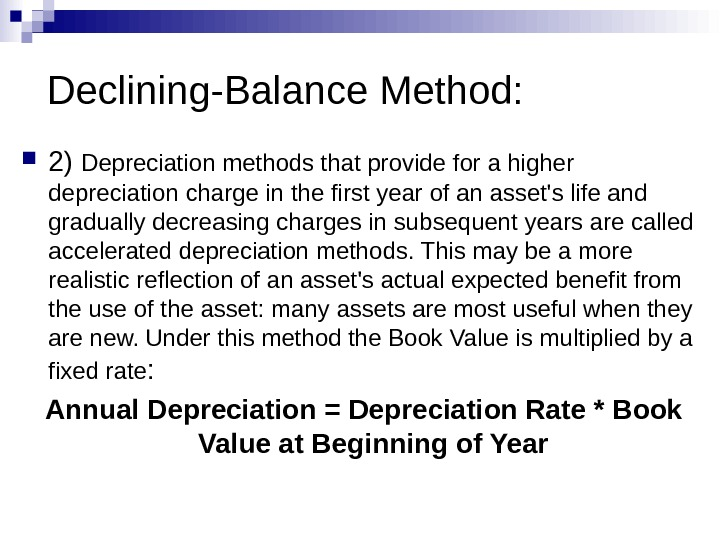Declining-Balance Method: 2) Depreciation methods that provide for a higher depreciation charge in the first year of an asset’s life and gradually decreasing charges in subsequent years are called accelerated depreciation methods. This may be a more realistic reflection of an asset’s actual expected benefit from the use of the asset: many assets are most useful when they are new. Under this method the Book Value is multiplied by a fixed rate : Annual Depreciation = Depreciation Rate * Book Value at Beginning of Year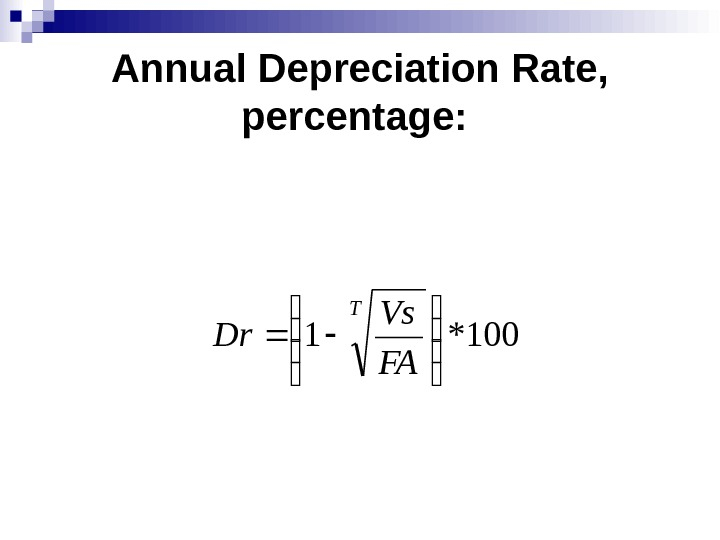Annual Depreciation Rate, percentage: 100*1 T FA Vs Dr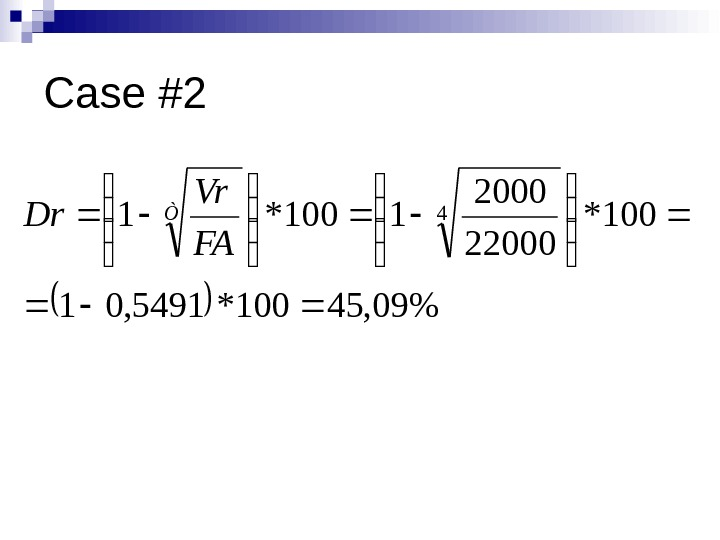Case #2 %09, 45100*5491, 01 100* 22000 1100*1 4 Ò FA Vr Dr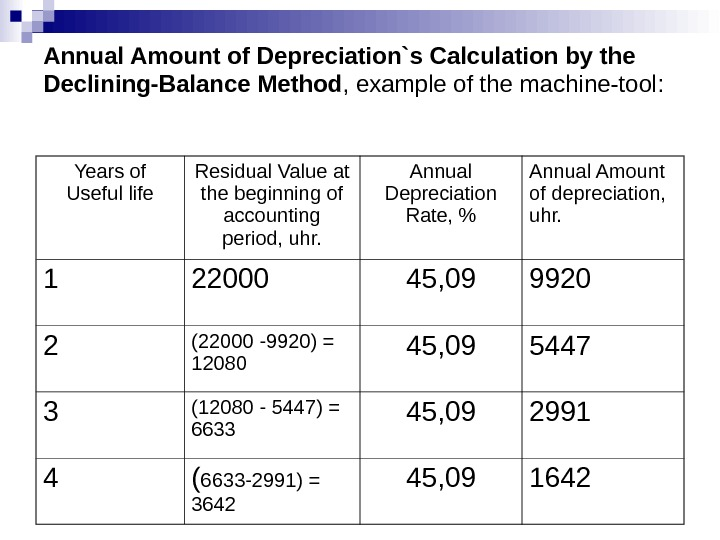Annual Amount of Depreciation`s Calculation by the Declining-Balance Method , example of the machine-tool: Years of Useful life Residual Value at the beginning of accounting period, uhr. Annual Depreciation Rate , % Annual Amount of depreciation, uhr. 1 22000 45, 09 9920 2 (22000 -9920) = 12080 45, 09 5447 3 (12080 — 5447) = 6633 45, 09 2991 4 ( 6633 -2991) = 3642 45,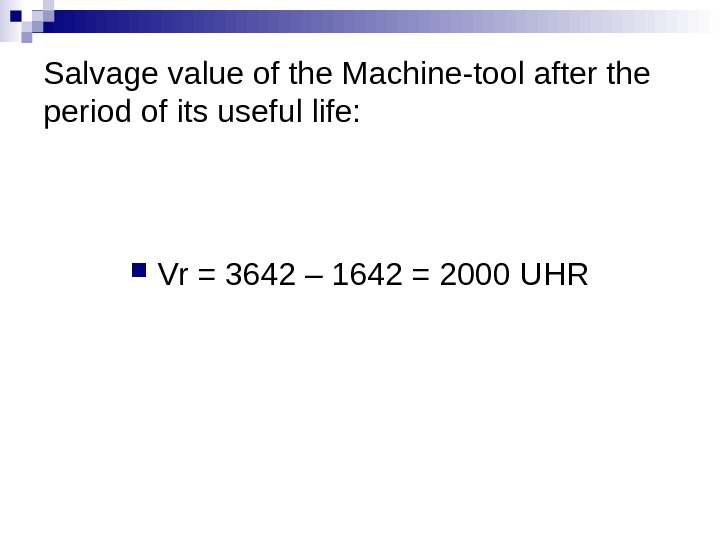Salvage value of the Machine-tool after the period of its useful life: Vr = 3642 – 1642 = 2000 UHR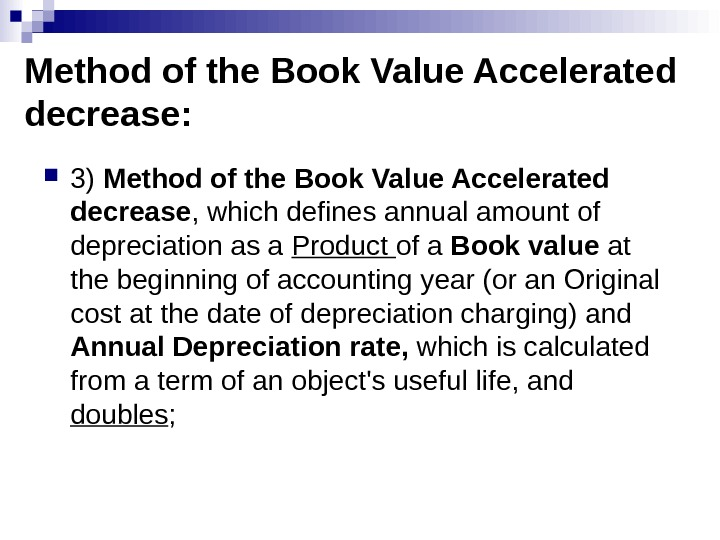Method of the Book Value Accelerated decrease: 3) Method of the Book Value Accelerated decrease , which defines annual amount of depreciation as a Product of a Book value at the beginning of accounting year (or an Original cost at the date of depreciation charging) and Annual Depreciation rate, which is calculated from a term of an о bject’s useful life, and doubles ;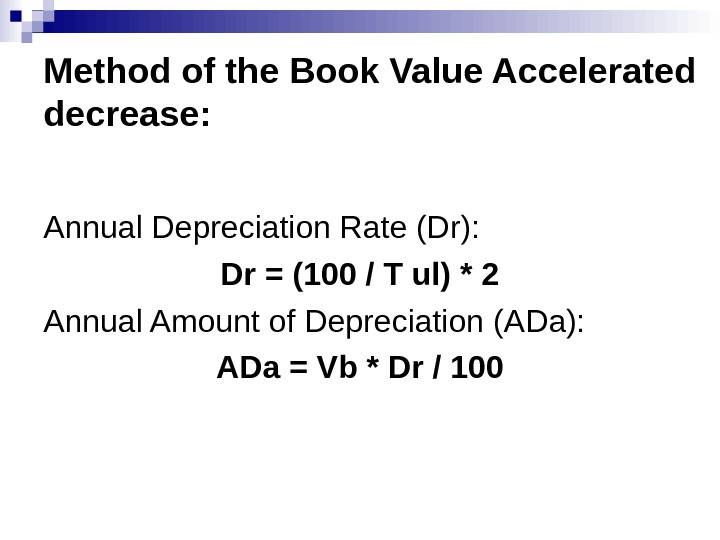Method of the Book Value Accelerated decrease: Annual Depreciation Rate (Dr) : Dr = (100 / T ul ) * 2 Annual Amount of Depreciation ( ADa ): ADa = Vb * Dr /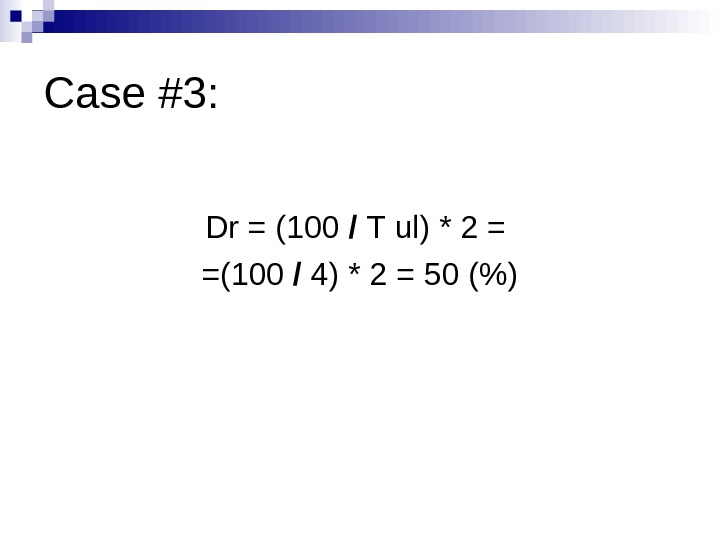Case #3: Dr = (100 / Т ul ) * 2 = = (100 / 4) * 2 = 50 (%)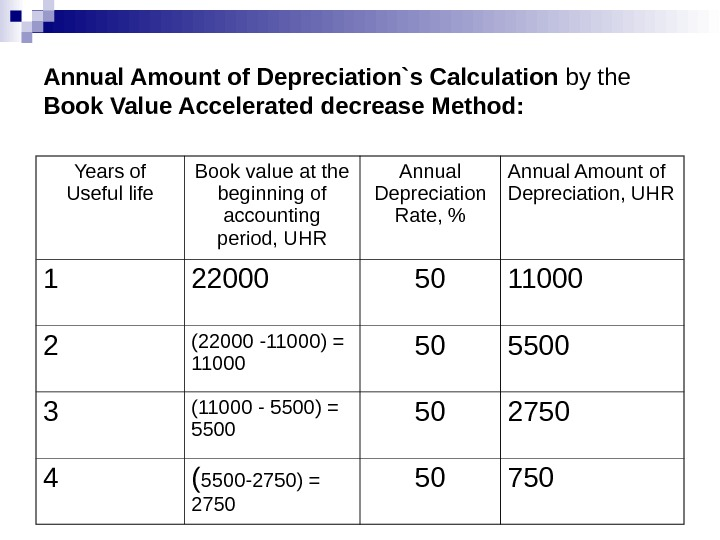Annual Amount of Depreciation`s Calculation by the Book Value Accelerated decrease Method: Years of Useful life Book value at the beginning of accounting period , UHR Annual Depreciation Rate, % Annual Amount of Depreciation, UHR 1 22000 50 11000 2 (22000 -11000) = 11000 50 5500 3 (11000 — 5500) = 5500 50 2750 4 ( 5500 -2750) =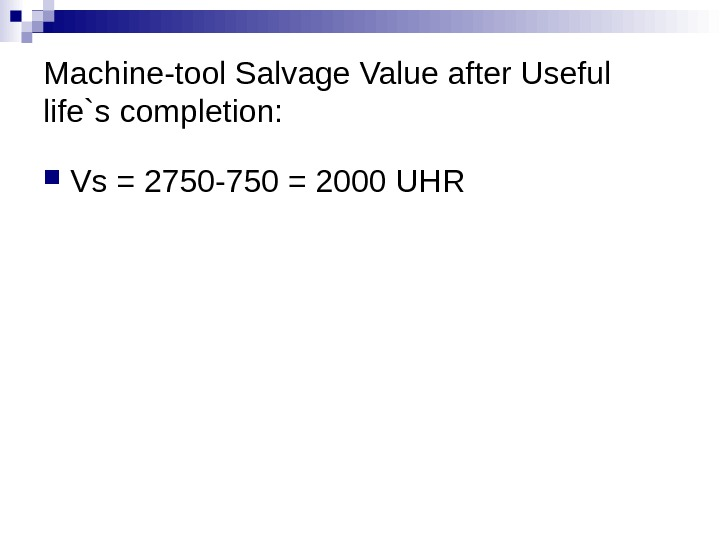Machine-tool Salvage Value after Useful life`s completion: Vs = 2750 -750 = 2000 UHR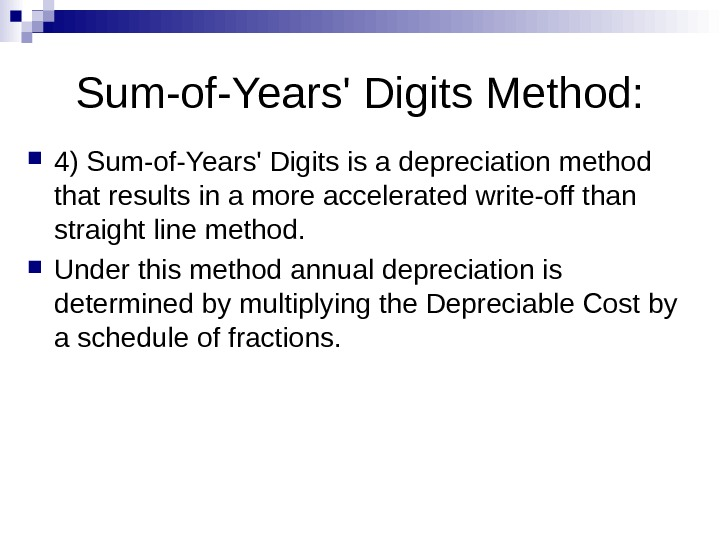Sum-of-Years’ Digits Method: 4) Sum-of-Years’ Digits is a depreciation method that results in a more accelerated write-off than straight line method. Under this method annual depreciation is determined by multiplying the Depreciable Cost by a schedule of fractions.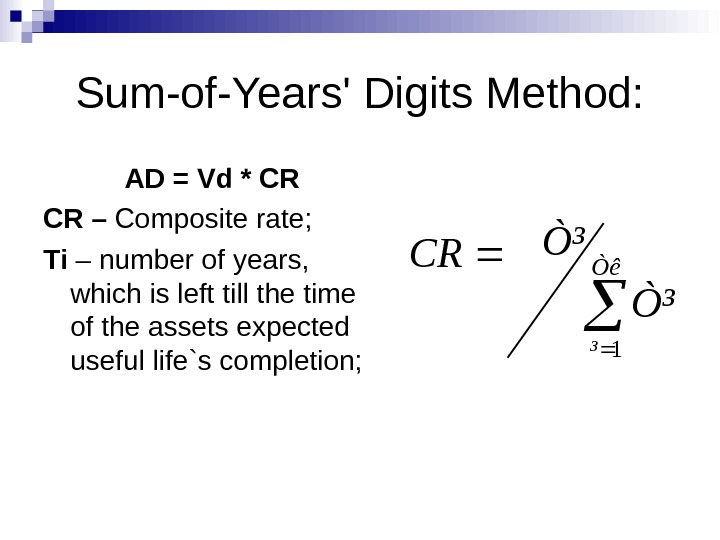Sum-of-Years’ Digits Method: AD = Vd * CR CR – Composite rate ; Ті – number of years, which is left till the time of the assets expected useful life`s completion; Òê ³ Ò³ Ò³ CR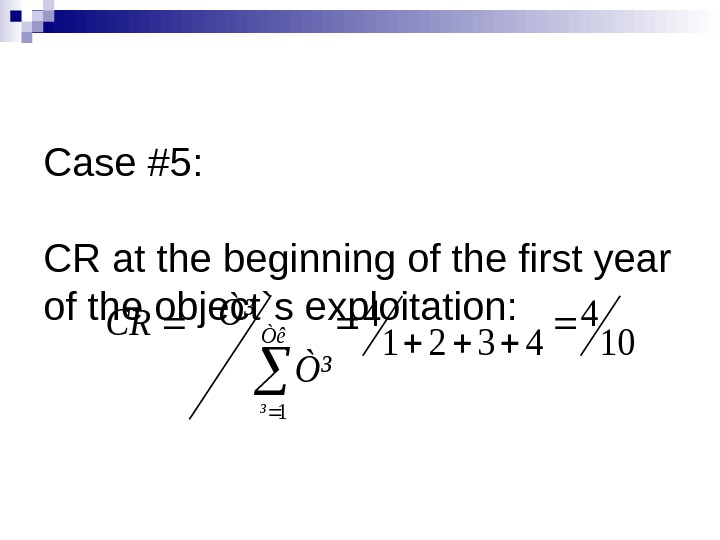Case #5 : CR at the beginning of the first year of the object`s exploitation: 10 4 4321 4 1 Òê ³ Ò³ Ò³ CR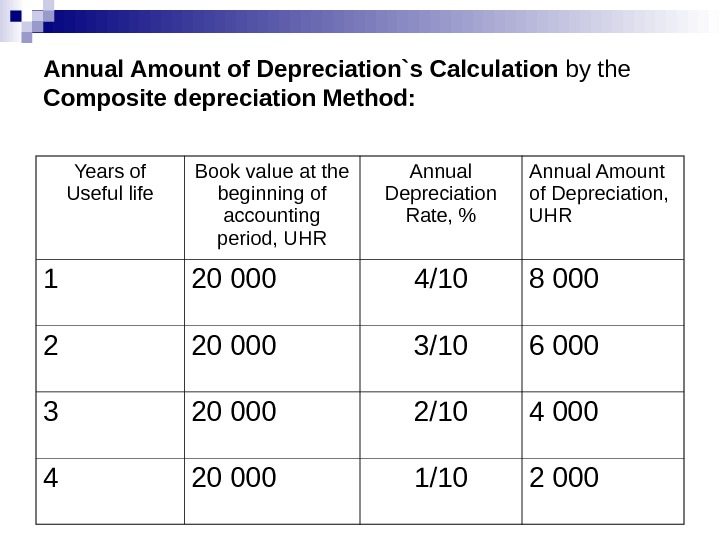Annual Amount of Depreciation`s Calculation by the Composite depreciation Method: Years of Useful life Book value at the beginning of accounting period , UHR Annual Depreciation Rate, % Annual Amount of Depreciation, UHR 1 20 000 4 /10 8 000 2 20 000 3/10 6 0 00 3 20 000 2/10 4 00 0 4 20 000 1/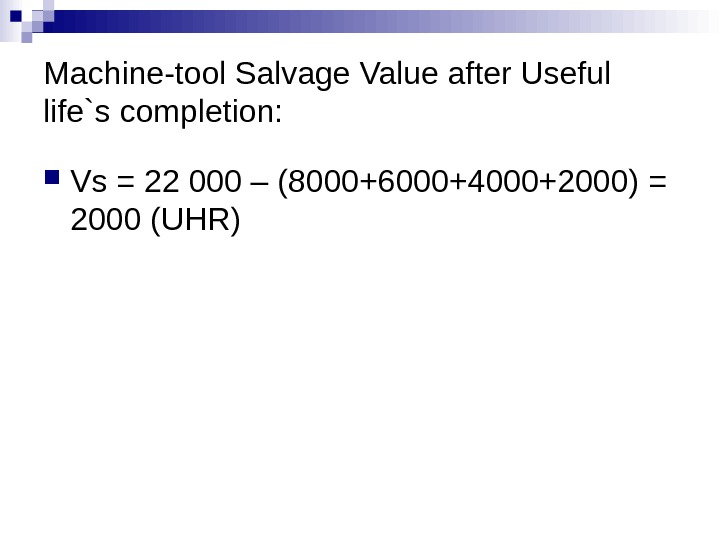Machine-tool Salvage Value after Useful life`s completion: Vs = 22 000 – (8000+6000+4000+2000) = 2000 ( UHR )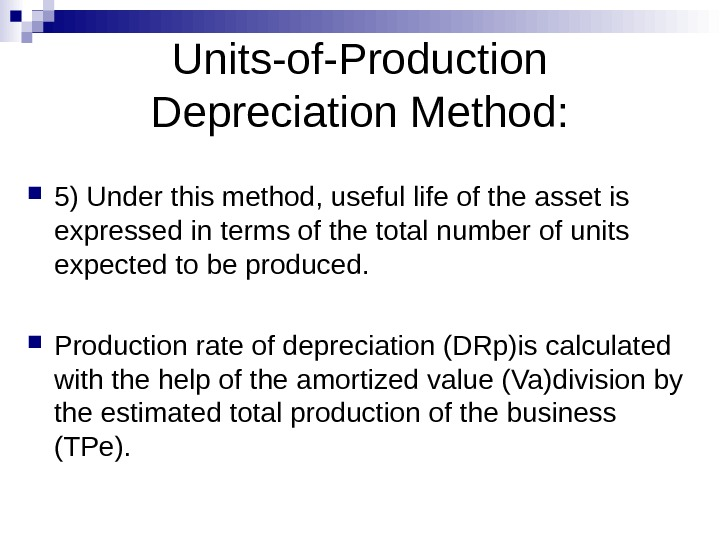Units-of-Production Depreciation Method: 5) Under this method, useful life of the asset is expressed in terms of the total number of units expected to be produced. Production rate of depreciation (DRp)is calculated with the help of the amortized value (Va)division by the estimated total production of the business (TPe).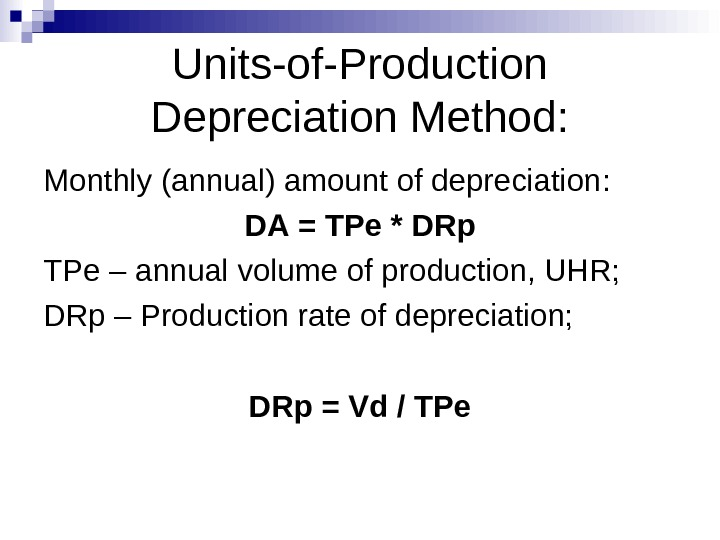Units-of-Production Depreciation Method: Monthly (annual) amount of depreciation : DA = TPe * DRp TPe – annual volume of production, UHR; DRp – Production rate of depreciation; DRp = Vd / TPe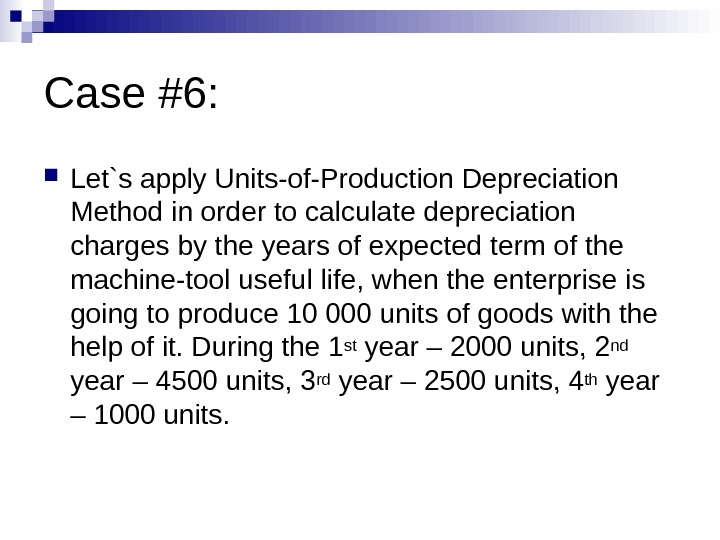Case #6: Let`s apply Units-of-Production Depreciation Method in order to calculate depreciation charges by the years of expected term of the machine-tool useful life, when the enterprise is going to produce 10 000 units of goods with the help of it. During the 1 st year – 2000 units, 2 nd year – 4500 units, 3 rd year – 2500 units, 4 th year – 1000 units.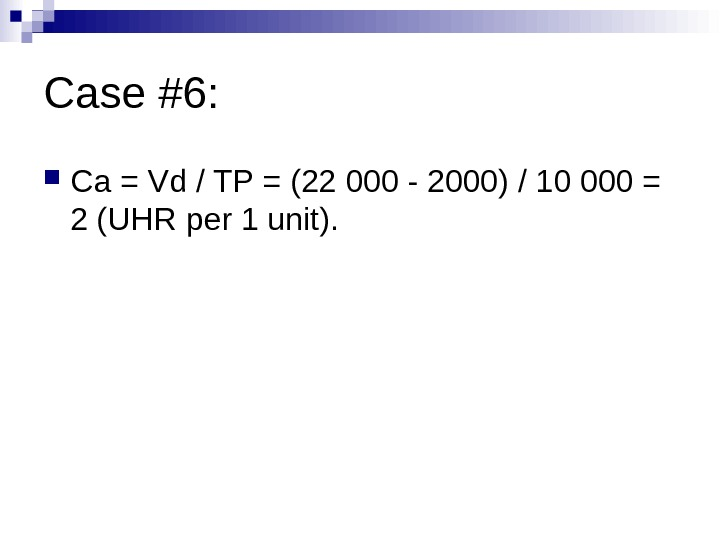Case #6: Са = Vd / TP = (22 000 — 2000) / 10 000 = 2 ( UHR per 1 unit ).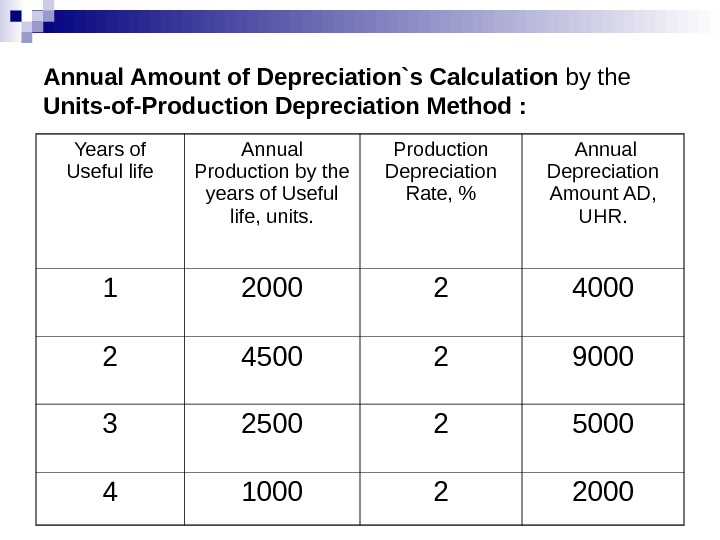Annual Amount of Depreciation`s Calculation by the Units-of-Production Depreciation Method : Years of Useful life Annual Production by the years of Useful life, units. Production Depreciation Rate , % Annual Depreciation Amount AD , UHR.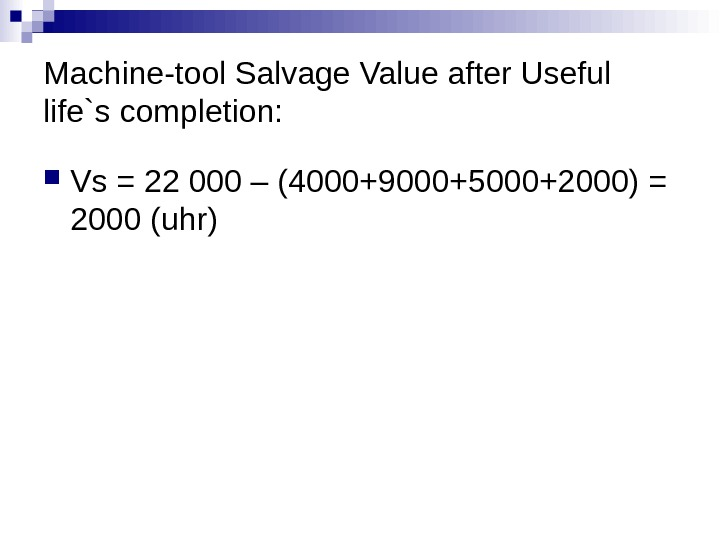Machine-tool Salvage Value after Useful life`s completion: Vs = 22 000 – ( 4 000+ 9 000+ 5 000+2000) = 2000 ( uhr )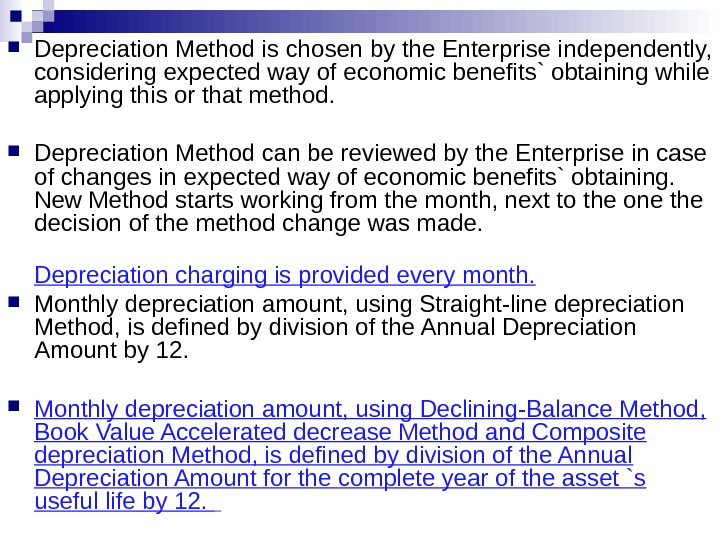Depreciation Method is chosen by the Enterprise independently, considering expected way of economic benefits` obtaining while applying this or that method. Depreciation Method can be reviewed by the Enterprise in case of changes in expected way of economic benefits` obtaining. New Method starts working from the month, next to the one the decision of the method change was made. Depreciation charging is provided every month. Monthly depreciation amount, using Straight-line depreciation Method, is defined by division of the Annual Depreciation Amount by 12. Monthly depreciation amount, using Declining-Balance Method, Book Value Accelerated decrease Method and Composite depreciation Method, is defined by division of the Annual Depreciation Amount for the complete year of the asset `s useful life by 12.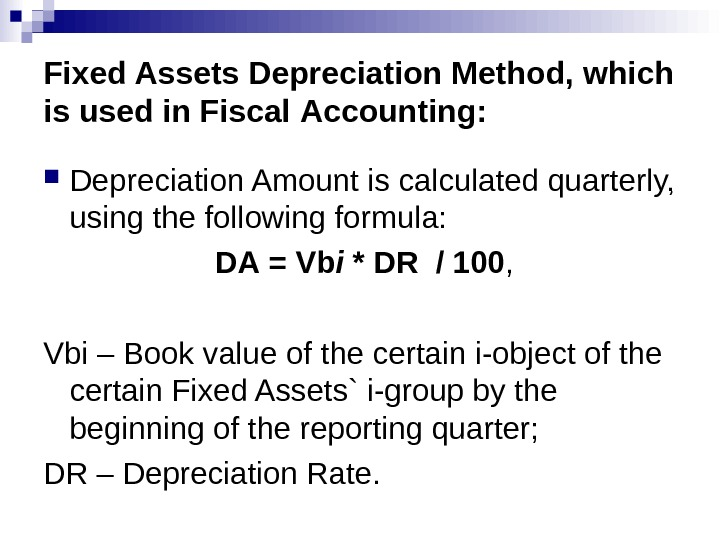Fixed Assets Depreciation Method, which is used in Fiscal Accounting: Depreciation Amount is calculated quarterly, using the following formula: DA = Vb і * DR / 100 , Vb і – Book value of the certain i-object of the certain Fixed Assets` i-group by the beginning of the reporting quarter; DR – Depreciation Rate.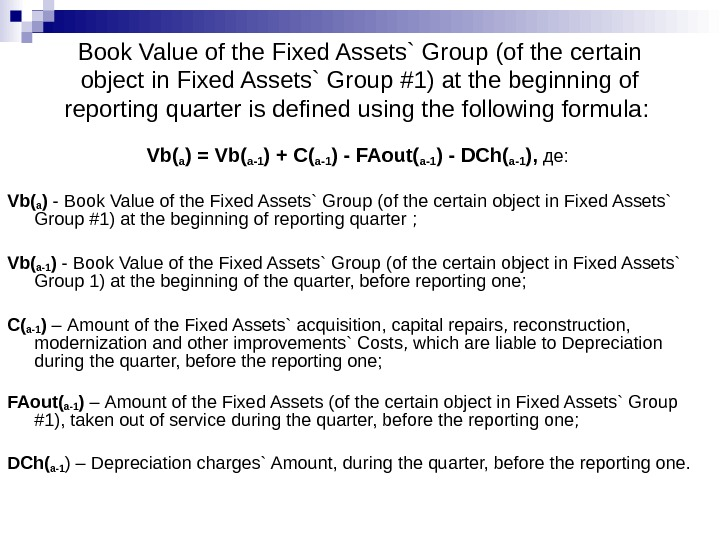Book Value of the Fixed Assets` Group (of the certain object in Fixed Assets` Group #1) at the beginning of reporting quarter is defined using the following formula : Vb ( а ) = Vb ( а-1 ) + C ( а-1 ) — FAout ( а-1 ) — DCh ( а-1 ), де: Vb (а ) — Book Value of the Fixed Assets` Group (of the certain object in Fixed Assets` Group #1) at the beginning of reporting quarter ; Vb ( а-1 ) — Book Value of the Fixed Assets` Group (of the certain object in Fixed Assets` Group 1) at the beginning of the quarter, before reporting one ; C ( а-1 ) – Amount of the Fixed Assets` acquisition, capital repairs, reconstruction, modernization and other improvements` Costs , which are liable to Depreciation during the quarter, before the reporting one; FAout ( а-1 ) – Amount of the Fixed Assets (of the certain object in Fixed Assets` Group #1), taken out of service during the quarter, before the reporting one; DCh ( а-1 ) – Depreciation charges` Amount, during the quarter, before the reporting one.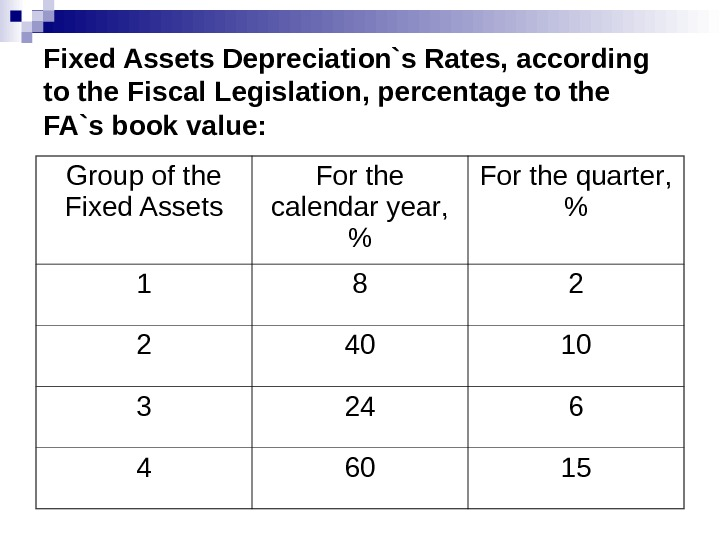Fixed Assets Depreciation`s Rates, according to the Fiscal Legislation, percentage to the FA`s book value: Group of the Fixed Assets For the calendar year , % For the quarter , %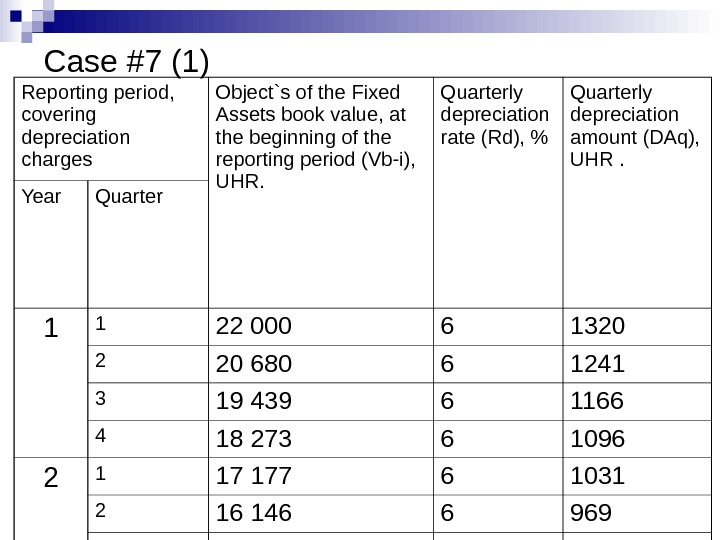Case #7 (1) Reporting period , covering depreciation charges Object`s of the Fixed Assets book value, at the beginning of the reporting period (Vb-i), UHR. Quarterly depreciation rate (Rd), % Quarterly depreciation amount (DAq), UHR . Year Quarter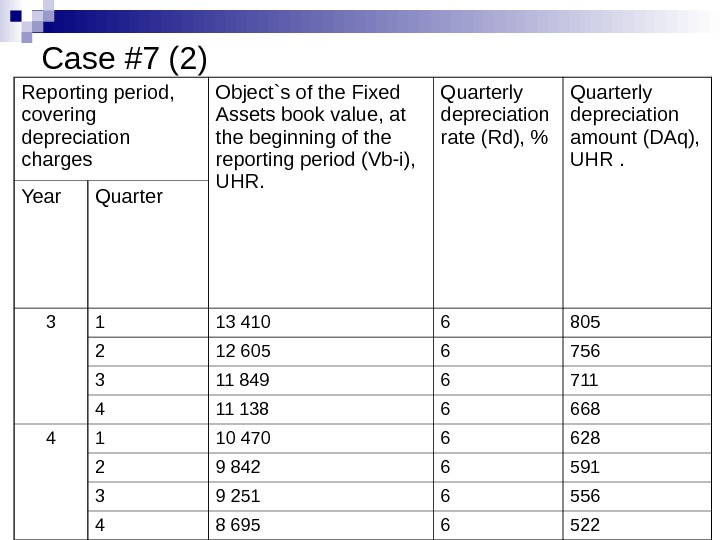Case #7 (2) Reporting period , covering depreciation charges Object`s of the Fixed Assets book value, at the beginning of the reporting period (Vb-i), UHR. Quarterly depreciation rate (Rd), % Quarterly depreciation amount (DAq), UHR . Year Quarter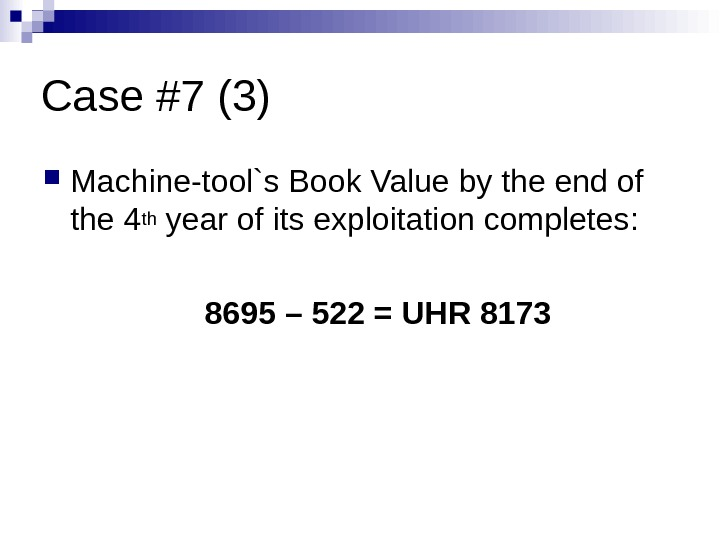Case #7 (3) Machine-tool`s Book Value by the end of the 4 th year of its exploitation completes : 8695 – 522 = UHR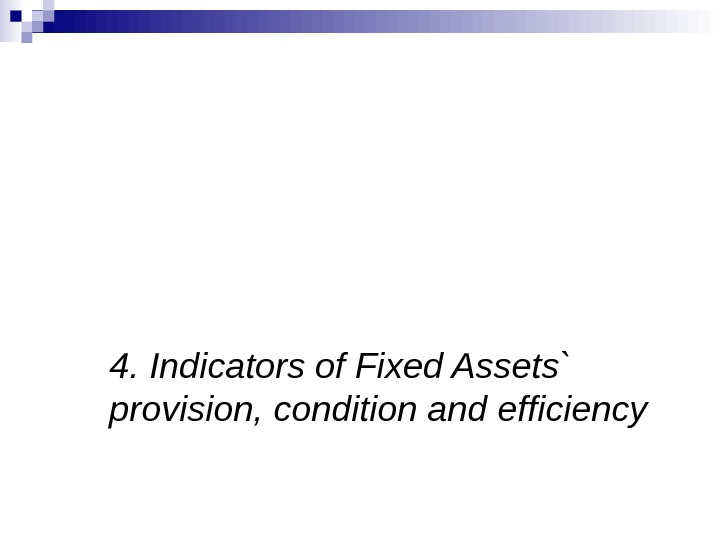4. Indicators of Fixed Assets` provision, condition and efficiencyIndicators of provision with the Fixed Assets : Capital intensity indicator (uhr/uhr) : CI = FAva / Q FAva – average value of the fixed assets during the certain period (quarter, year) ; Q – Volume of production during the same period.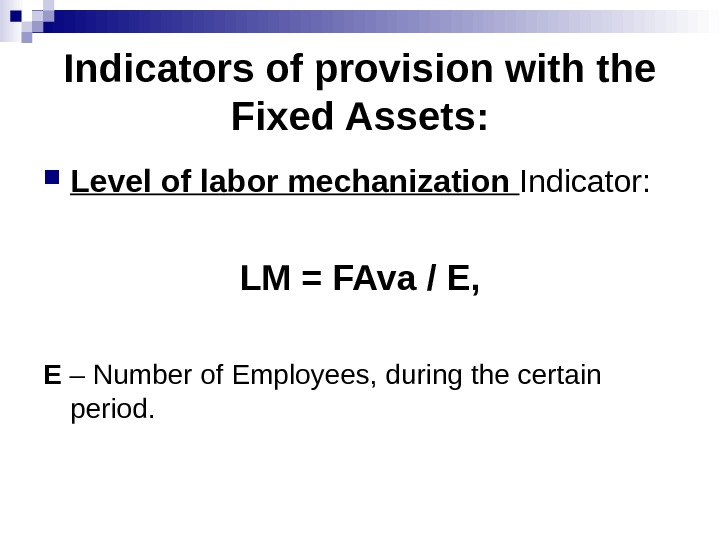Indicators of provision with the Fixed Assets : Level of labor mechanization Indicator: LM = FAva / E, E – Number of Employees, during the certain period.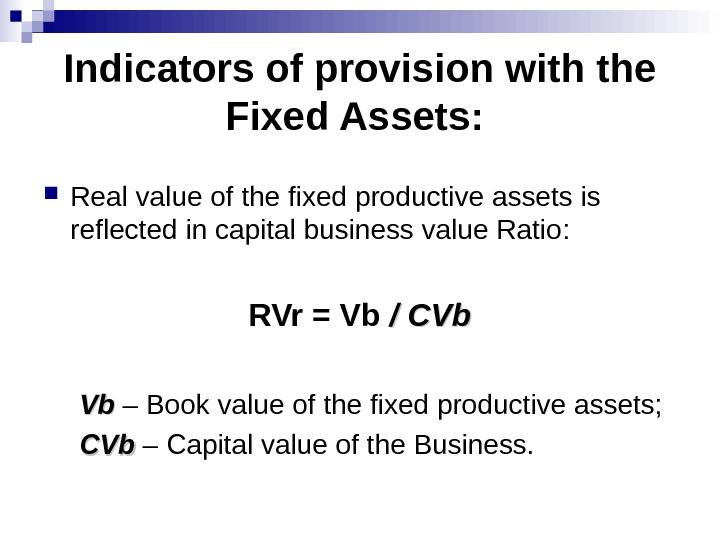Indicators of provision with the Fixed Assets : Real value of the fixed productive assets is reflected in capital business value Ratio : RVr = Vb / CVb Vb. Vb – Book value of the fixed productive assets; CVb – Capital value of the Business.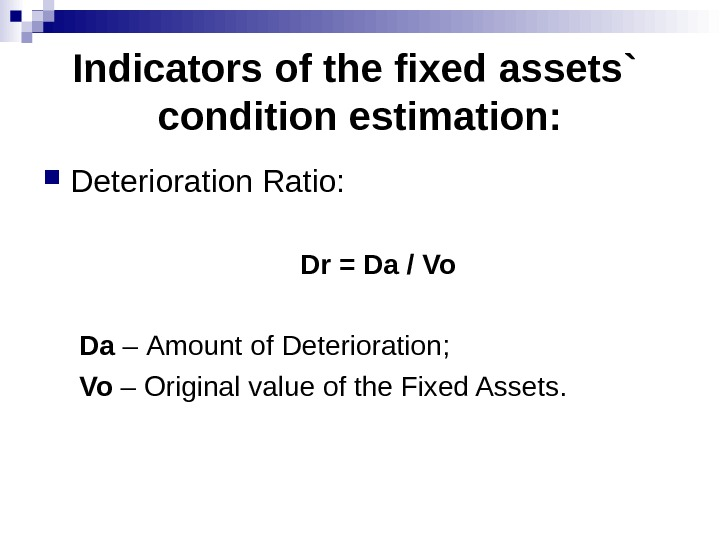Indicators of the fixed assets` condition estimation: Deterioration Ratio : Dr = Da / Vo Da – Amount of Deterioration ; Vo – Original value of the Fixed Assets.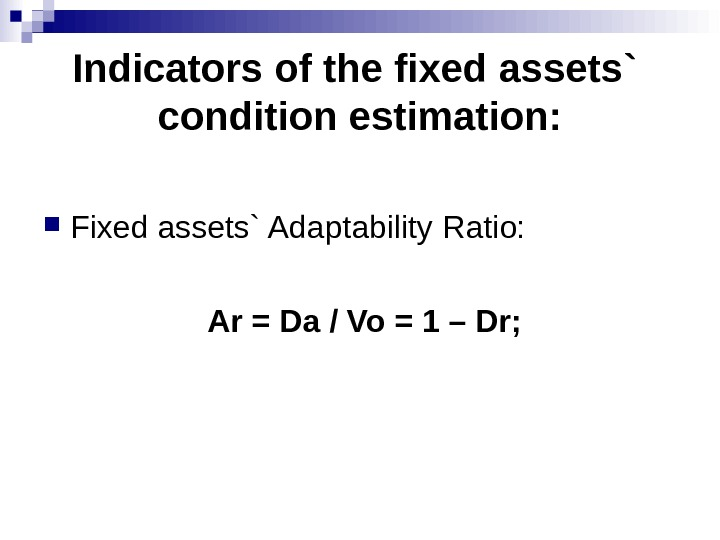Indicators of the fixed assets` condition estimation: Fixed assets` Adaptability Ratio: Ar = Da / Vo = 1 – Dr;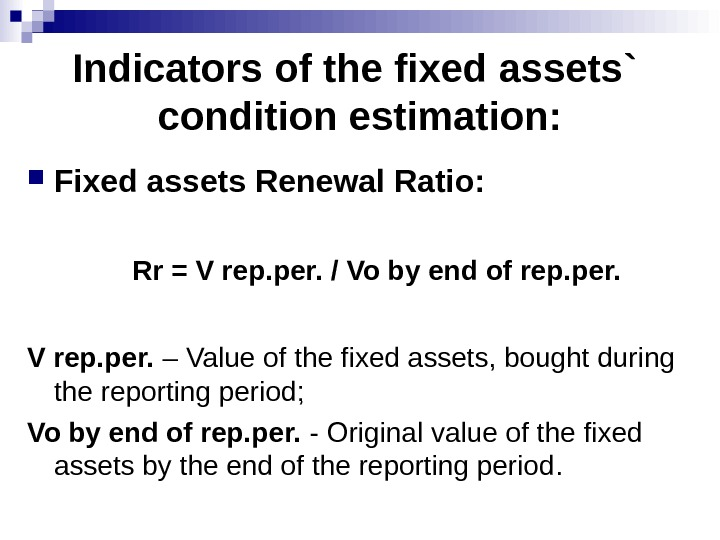Indicators of the fixed assets` condition estimation: Fixed assets Renewal Ratio : Rr = V rep. per. / Vo by end of rep. per. V rep. per. – Value of the fixed assets, bought during the reporting period ; Vo by end of rep. per. — Original value of the fixed assets by the end of the reporting period.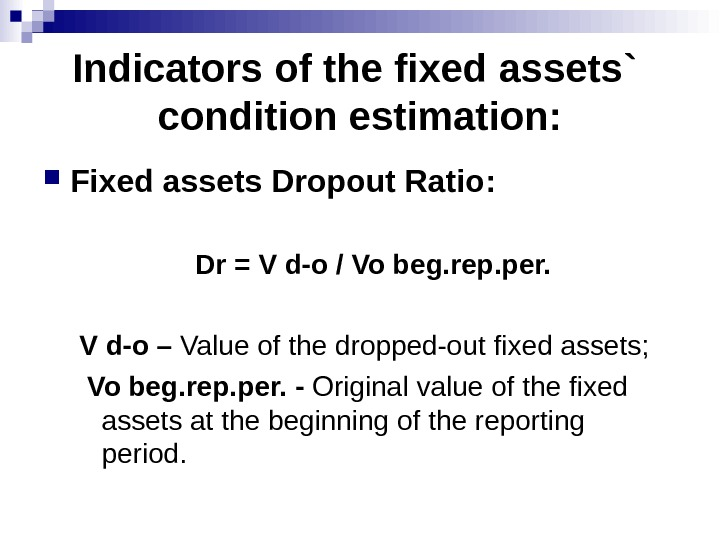Indicators of the fixed assets` condition estimation: Fixed assets Dropout Ratio : Dr = V d-o / Vo beg. rep. per. V d-o – Value of the dropped-out fixed assets ; Vo beg. rep. per. — Original value of the fixed assets at the beginning of the reporting period.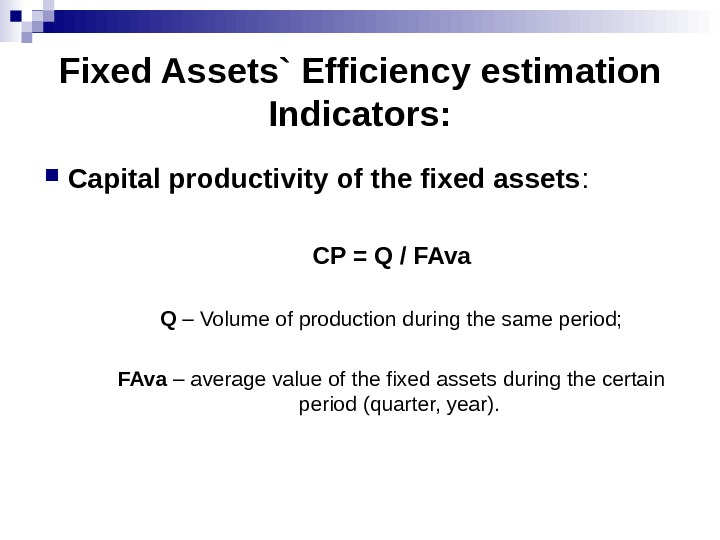Fixed Assets` Efficiency estimation Indicators: Capital productivity of the fixed assets : CP = Q / FAva Q – Volume of production during the same period; FAva – average value of the fixed assets during the certain period (quarter, year).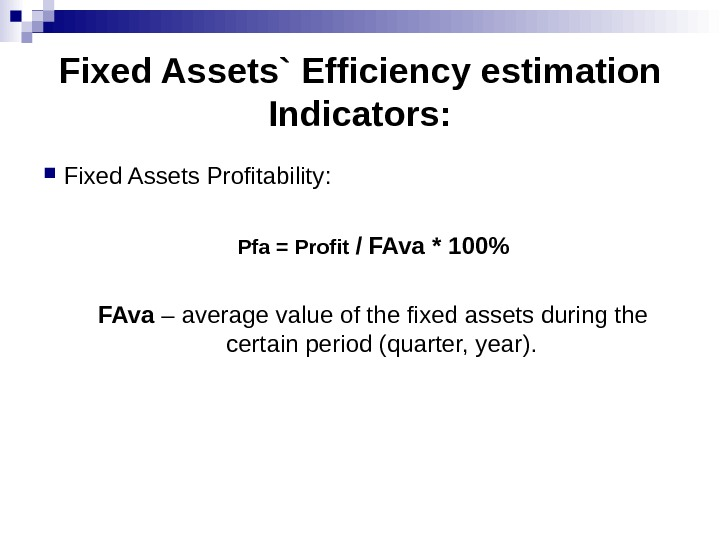Fixed Assets` Efficiency estimation Indicators: Fixed Assets Profitability : Pfa = Profit / FAva * 100% FAva – average value of the fixed assets during the certain period (quarter, year).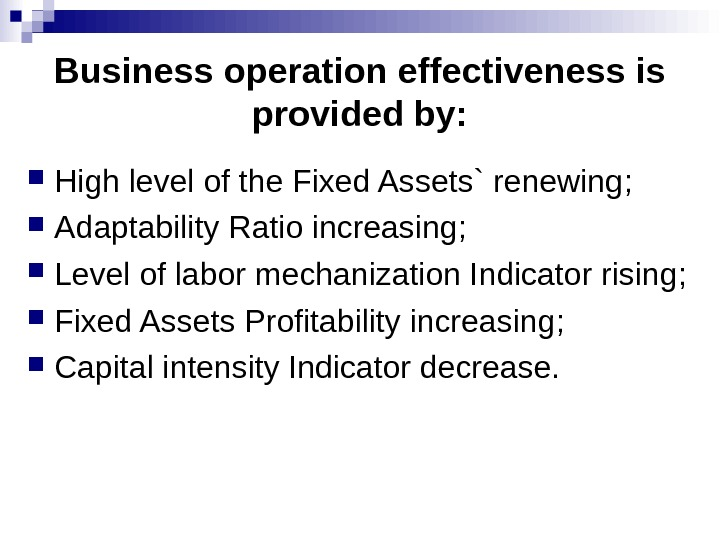Business operation effectiveness is provided by : High level of the Fixed Assets` renewing ; Adaptability Ratio increasing ; Level of labor mechanization Indicator rising ; Fixed Assets Profitability increasing ; Capital intensity Indicator decrease.